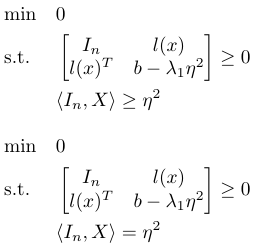# How to fix the constraint

Hi, this is my first time here.

I confront a simple problem:

clear all;
close all;
clc
A = [1 2;
1 0];

cvx_begin sdp
variables x(2)
minimize(0)
subject to
[eye(2) Ax;(Ax)’ 7]>=0;
norm(x,2)<=2;
cvx_end

The original constraint is: <I_2, X> = 4

1. < > is a matrix inner product.
2. X = xx^T

I try to use norm(x,2) == 2; however, it is an error.
norm(x,2)<=2 is correct; however, the optimal solution is [0;0], which is not what I want.

I try to use
norm(x,2)<=2.01;
norm(x,2)>=1.99;

it shows:
Invalid constraint: {convex} >= {real constant}

How do I fix it?

norm(x) is a convex function, so a constraint
norm(x) <= real constant
is convex and follows CVX 'x DCP rules.

norm(x) >= real constant
and
norm(x) == real constant
are not convex and are not allowed n CVX.

So your problem as described is non-convex.

However, because you have specified an objective
minimize(0)
you just have a feasibility problem, which means you could try to use a convex objective to help you out. I played around for a minute with different objective functions, and discovered that minimize(x(2)) or maximize(x(2)) produce a solution for which norm(x) = 2, and therefore satisfy your original feasibility problem.

cvx_begin sdp
variables x(2)
maximize(x(2))
subject to
[eye(2) A*x;(A*x)' 7]>=0;
norm(x,2)<=2;
cvx_end

Calling SeDuMi 1.34: 10 variables, 3 equality constraints
For improved efficiency, SeDuMi is solving the dual problem.
------------------------------------------------------------


Edited out solver output
------------------------------------------------------------
Status: Solved
Optimal value (cvx_optval): +1.72721

>> disp(x)
-1.008345327824377
1.727205748021872
>> disp(norm(x))
1.999999999036261


Changing the objective function to minimize(x(2)) results in the optimal x being the negative of that above, and so is another feasible solution. I don’t claim that these are the only feasible solutions, but given that you specified a feasibility problem, even if it had been convex and allowed by CVX, CVX would have only provided you a single feasible solution.

1 Like

Thanks!!

However, if the problem becomes more general such as the following (2 cases):Note:

1. X=xx^T, where x \in R^n
2. l(x) \in R^n. Each element in this vector is a polynomial of x_i . Yes, the first inequality is an LMI.
3. In is an identity matrix.

How to rewrite the second inequality as a valid cvx inequality constraint?

It is equivalent to ||x||_2^2 =\eta^2 or ||x||_2^2 \geq \eta^2 then how to fix it in a more general way?

The problem is non-convex. I don’t see how to solve it in a general way with CVX.

For your first version, maybe I was just lucky to stumble upon a solution, or as I was thinking, maybe the RHS value of 2 was not "random’, and so something was going on which made that work out. Specifically, the optimal x was was just on the boundary of feasibility of the LMI, i.e., minimum LHS eigenvalue = 0, and that corresponded to norm(x) = 2.

1 Like

I’m certain this FAQ has reduced the number of questions like this on the forum. And still: 90% of the posts we mark as Nonconvex are fully addressed by it.

1 Like